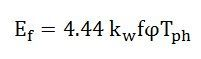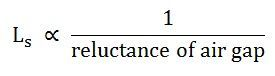# Short Circuit Ratio of a Synchronous Machine

The Short Circuit Ratio (SCR) of a synchronous machine is defined as the ratio of the field current required to generate rated voltage on an open circuit to the field current required to circulate rated armature current on a short circuit. The short circuit ratio can be calculated from the open-circuit characteristic ( O.C.C) at rated speed and the short circuit characteristic (S.C.C) of a three-phase synchronous machine as shown in the figure below:From the above figure, the short circuit ratio is given by the equation shown below.Since the triangles Oab and Ode are similar. Therefore,The direct axis synchronous reactance Xd is defined as the ratio of open-circuit voltage for a given field current to the armature short circuit current for the same field current.

For the field current equal to Oa, the direct axis synchronous reactance in ohms is given by the equation shown below:From equation (1) and equation (6), we getFrom equation (7) it is clear that the short circuit ratio is equal to the reciprocal of the per-unit value of the direct axis synchronous reactance.

In a saturated magnetic circuit, the value of Xd depends upon the degree of saturation.

## Significance of Short Circuit Ratio (SCR)

Short Circuit Ratio is an important factor of the synchronous machine. It affects the operating characteristics, physical size, and cost of the machine. The large variation in the terminal voltage with a change in load takes place for the lower value of the short circuit ratio of a synchronous generator. To keep the terminal voltage constant, the field current (If) has to be varied over a wide range.

For the small value of the short circuit ratio (SCR), the synchronizing power is small. As the synchronizing power keeps the machine in synchronism, a lower value of the SCR has a low stability limit. In other words, a machine with a low SCR is less stable when operating in parallel with the other generators.

A synchronous machine with a high value of SCR had a better voltage regulation and improved steady-state stability limit, but the short circuit fault current in the armature is high. It also affects the size and cost of the machine.

The excitation voltage of the synchronous machine is given by the equation:For the same value of Tph Excitation voltage is directly proportional to the field flux per pole.The synchronous inductance is given as:Hence, the short circuit ratio is directly proportional to the air gap reluctance or air gap length.

If the length of the air gap is increased, the SCR can be increased. With the increase in the air gap length, the field MMF is to be increased for the same value of excitation voltage (Ef). Hence, to increase the value of field MMF either field current or the number of field turns has to be increased. All this requires a greater height of field poles and, as a result, the overall diameter of the machine increases.

Thus, a conclusion is that the large value of SCR will increase the size, weight, and cost of the machine.

The typical values of the SCR for different types of machines are as follows:-

• For cylindrical rotor machines, the value of SCR lies between 0.5 to 0.9.
• In the case of the Salient-pole machine, it lies between 1 to 1.5 and
• For synchronous compensators, it is 0.4.

This is all about Short Circuit Ratio of a Synchronous Machine.

### 12 thoughts on “Short Circuit Ratio of a Synchronous Machine”

1. SUBRAMONIAM SIVARAMAKRISHNAN

Nice explanation. Kept it simple and even after 31 years being out of touch with machines I could recap the concept easily.